## Miquel's Theorem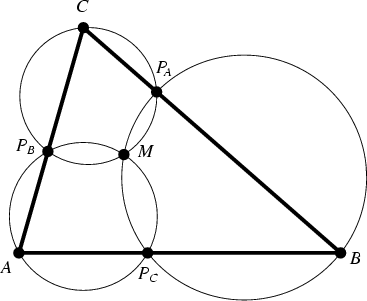If a point is marked on each side of a Triangle, then the three Miquel Circles (each through a Vertex and the two marked points on the adjacent sides) are Concurrent at a point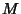called the Miquel Point. This result is a slight generalization of the so-called Pivot Theorem.

Iflies in the interior of the triangle, then it satisfies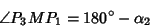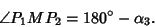The lines from the Miquel Point to the marked points make equal angles with the respective sides. (This is a by-product of the Miquel Equation.)Given four lines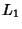, ...,each intersecting the other three, the four Miquel Circles passing through each subset of three intersection points of the lines meet in a point known as the 4-Miquel point. Furthermore, the centers of these four Miquel Circles lie on a Circle(Johnson 1929, p. 139). The lines fromto given points on the sides make equal Angles with respect to the sides.

Similarly, givenlines taken by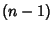s yieldMiquel Circles likepassing through a point, and their centers lie on a Circle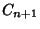.

See also Miquel Circles, Miquel Equation, Miquel Triangle, Nine-Point Circle, Pedal Circle, Pivot Theorem

References

Johnson, R. A. Modern Geometry: An Elementary Treatise on the Geometry of the Triangle and the Circle. Boston, MA: Houghton Mifflin, pp. 131-144, 1929.